Latest Banking jobs   »   Quantitative Aptitude Quiz For SBI Clerk...

# Quantitative Aptitude Quiz For SBI Clerk Mains exam 2021- 15th August

Q1. Mr. Suresh opened a workshop investing Rs. 40000. He invested additional amount of Rs. 12000 at the end of every year. After 2 years his brother Ramesh joined him with an amount of Rs. 85000. Thereafter Ramesh did not invested any additional amount. Find the difference between their shares in profit, if the sum of their profit at the end of 4 years is Rs. 603000.
(a) 91000 Rs.
(b) 95000 Rs.
(c) 80000 Rs.
(d) 93000 Rs.
(e) 83000 Rs.

Q2. A man goes downstream with a boat to some destination and returns upstream to his original place in 5 hours. If the speed of the boat in still water and the speed of stream are 10km/h and 4km/h respectively, the distance of the destination from the starting place is:
(a)21.5 km
(b) 22 km
(c) 20 km
(d) 21 km
(e) 18 km

Q3. A started a business, B and C joined him in the 1st year ,they invested in the ratio of 5:4:7 respectively and the period for which they invested was in the ratio of 4:3:2 respectively. In the 2nd year , A doubled the investment, B and C continued with the same investment as they investor for the same no. of month as they did in 1st year. The total profit after 2 years was 14000. What is B’s share of profit.?
(a) Rs 2500
(b) Rs 3000
(c) Rs 3500
(d) Rs 4000
(e) Rs 4500

Q4. Two buses, one moving towards North and the other towards South, leave the same place at the same time. The speed of one of them is greater than that of the other by 5 km/h. At the end of 2 hrs, they are at a distance of 50 km from each other. The speed of the bus going slower is
(a) 15 km/h
(b) 12 km/h
(c) 10 km/h
(d) 20 km/h
(e) 25 km/h

Q5. A train starts from Delhi at 6 : 00 a.m. and reaches Ambala Cantt at 10 a.m. The other train starts from Ambala Cantt at 8 a.m. and reaches Delhi at 11 : 30 a.m. If the distance between Delhi and Ambala Cantt is 200 km, then at what time did the two trains meet each other?
(a) 8 : 56 a.m.
(b) 8 : 46 a.m.
(c) 7 : 56 a.m.
(d) 8 : 30 a.m.
(e) 9 : 00 a.m.

Directions (6-10): The following questions are accompanied by three statements I or A, II or B and III or C. You have to determine which statement(s) is/are sufficient/necessary to answer the questions and mark your answer accordingly

Q6. What is the average age of the six members A, B, C, D, E& F in a family?
I. Total age of D & E is 14 years.
II. Average age of A, B, C and F is 50 years
III Average age of A, B, D and E is 40 years
(a) Only I & II
(b) Only I & III
(c) Only II & Ill
(d) All I, II & III
(e) None of these

Q7. What is the rate of interest p.a?
I. A sum doubles itself at simple interest in 10 years.
II. The difference between the compound interest and the simple interest on a sum of Rs. 15,000 in two years is Rs. 150.
III. The compound interest accrued in 8 years is more than the amount (principal).
(a) Only I
(b) Only II
(c) Only II & III
(d) Only I & III
(e) Only I or only II

Q8. 8 men and 6 women can complete a piece of work in 21 days. How many days will it take for 12 men and 9 women to complete the same work?
A. 6 men can complete the work in 42 days.
B. 7 women can complete the work in 63 days.
C. The amount of work done by a woman is four seventh of the work done by a man in one day.
(a) Any two of them
(b) Any of them
(c) Only C
(d) Either A or B only
(e) No need of any information

Q9. A train crosses another train in 10 sec. Find out the lengths of the trains.
A. Ratio between the lengths the of second and first train is 4 : 5.
B. Ratio between the speed of first and second trains is 1 : 2.
C. The speed of first train is 36 km/hr.
(a) Only A and B together
(b) Only B and C together
(c) Only A and C together
(d) Questions can’t be answered even after using all the information
(e) None of these

Q10. What is the cost price of a table?
A. By selling the table for Rs. 600 instead of Rs. 500, loss per cent decreases by 10%.
B. When the cost price of the table increases by 10% and then decreases by 10%, it reduces by Rs. 10.
C. By selling the table and a chair for Rs. 1500 a net profit of 25% is made.
(a) Only A or B alone
(b) Only B or C alone
(c) Only A and C together
(d) Any two of them together
(e) Either B alone or A and C together are sufficient

Directions (11-15): What approximate value comes in the place of question (?) mark: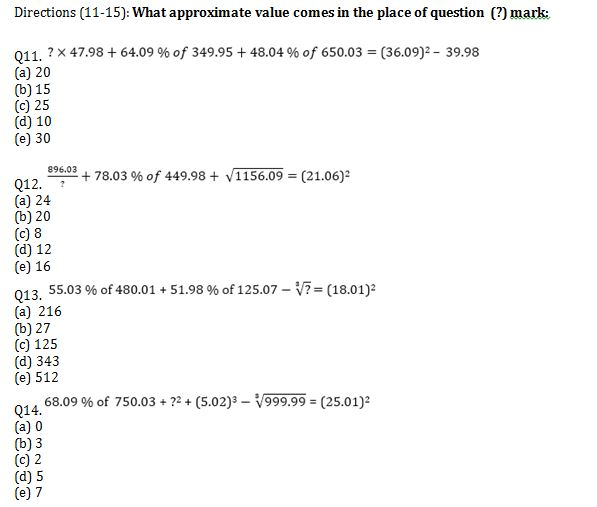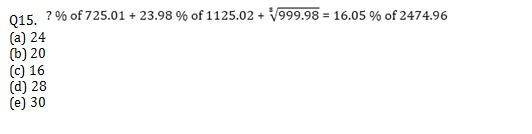Solutions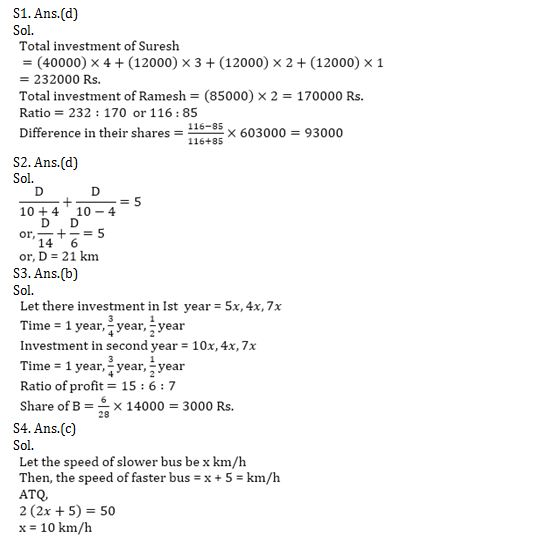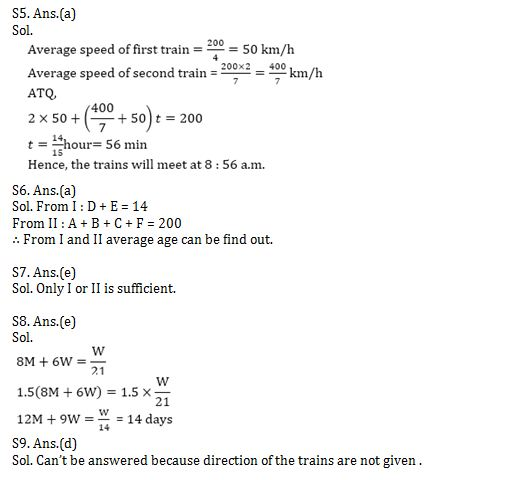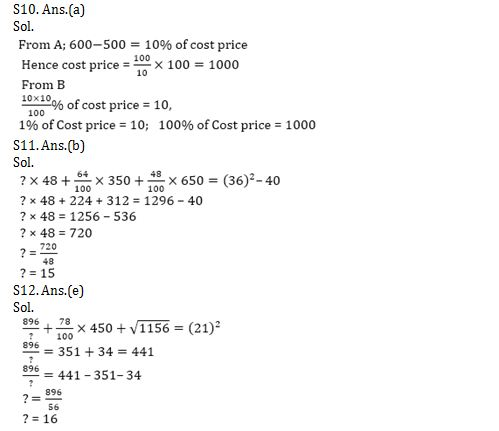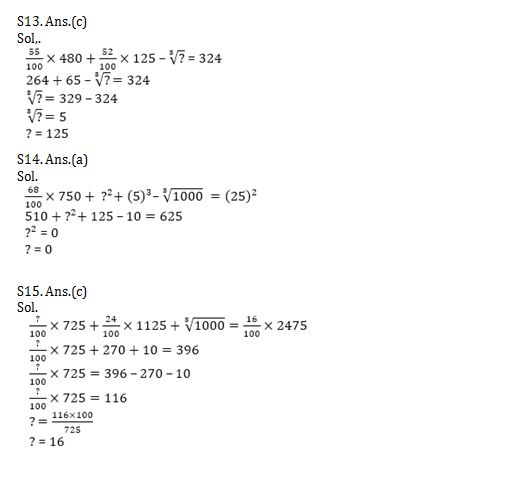#### Congratulations!Union Budget 2023-24: Free PDF